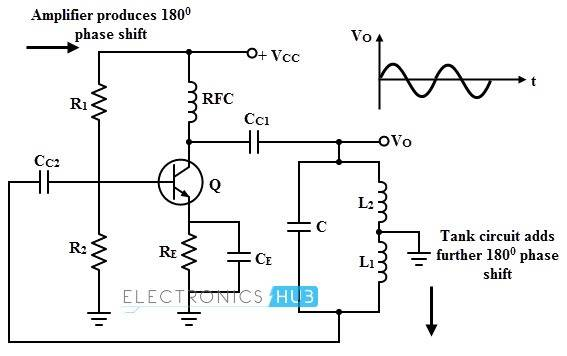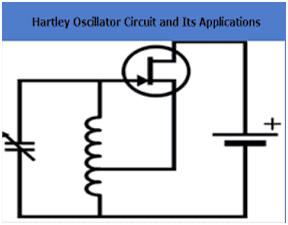# Accounting for mutual inductance in a Hartley Oscillator

• cianfa72

#### cianfa72

TL;DR Summary
About the calculation of equivalent inductance when taking into account mutual induction in the analysis of Hartley Oscillator
Hi,

I'm watching a video about Hartley oscillator and I'm in trouble with a simple assumption: as stated at minute 5:50 if the two coils ##L_1## and ##L_2## are wound on the same core then taking into account the mutual inductance M he gets:

##L^ {'} _1 = L_1 + M##
##L^ {'} _2 = L_2 + M##

From my understanding it surely makes sense if we assume equals the currents through the two coils ##I_{L_1} = I_{L_2} = I##.
However I believe they are not the same from the analysis of the small signal equivalent circuit at least when we do *not neglect* the BJT input impedance ##h_{ie}##.

Last edited:

One capacitor is connected between the ends of the inductors, so the currents will be equal if the capacitor current dominates the inductors. That will be the case with a high Q oscillator circuit.

You might also consider that the two inductors make a centre-tapped autotransformer, where the ratio of the turns count is equal to the ratio of the voltages. That will give the inversion, (180° phase shift), required for oscillation.

One capacitor is connected between the ends of the inductors, so the currents will be equal if the capacitor current dominates the inductors. That will be the case with a high Q oscillator circuit.
Thus basically what you are saying is that a good design of the circuit (high Q factor) implies we can *reasonably* assume ##I_{L_1} = I_{L_2} = I_C = I## ?

Yes. The tank is an LC resonant circuit that supports a circulating current.

In principle, there are two basic explanations how the Hartley oscillator works.
(1) The first one - as discussed already - starts with an LC tank circuit which (due to the grounded midpoint of the coil) provides (at resonance) a 180deg phase shift at both ends .
(2) Second explanation: The feedback path consists of a 4th-order highpass (C2-L2-C-L1) in ladder topology which - at one single frequency - also can provide a 180deg phase shift.

The basic explanation I found is the following -- see for instance https://www.electrical4u.com/hartley-oscillator/On switching ON the power supply, the transistor starts to conduct, leading to an increase in the collector current, Ic which charges the capacitor C. On acquiring the maximum charge feasible, C starts to discharge via the inductors L1 and L2. This charging and discharging cycles result in the damped oscillations in the tank circuit. The oscillation current in the tank circuit produces an AC voltage across the inductors L1 and L2 which are out of phase by 180deg as their point of contact is grounded.

My doubt is about the first part of the sentence: my understanding is that upon switching ON the power supply, RFC forces the current through it to stay at 0 -- before powering ON its flux was actually null. Then the current starts to increase (thanks to the differential resistance of collector-emitter) flowing into the collector and charging the capacitor C

If that's correct, why the capacitor C starts to discharge via inductors L1 and L2 only later when its maximum charge has been reached ?

Last edited:
.....
If that's correct, why the capacitor C starts to discharge via inductors L1 and L2 only later when its maximum charge has been reached ?
I think, itis not a simple matter to explain in the time domain the starting behavior of such a tank circuit (with ground connection between both inductors).
Therefore, I personally prefer the 4th-order highpass explanation.

•Baluncore
I think, itis not a simple matter to explain in the time domain the starting behavior of such a tank circuit (with ground connection between both inductors).
Therefore, I personally prefer the 4th-order highpass explanation.
Referring to the schematic in post #6, does the 4th-order highpass filter consist of Cc1-L2-C-L1 ?

There are several ways of drawing a Hartley oscillator. The usual way I have seen is given like this and is a very convenient arrangement because no RFC is required, one side of the tuning capacitor is grounded (avoiding hand-capacitance) and no RFC is required. Of course, bias and decoupling components will be required but their reactance should be negligible. It is not usual to employ these components as part of the LC network, to create a ladder network for instance.In the configuration shown there are only two frequency determining components. I think the distinction from the Colpitts circuit is that the latter uses a capacitive tap. A capacitive tap is equivalent to a resonant transfomer, where the turns ratio corresponds to the capacitor ratio. The transformer shown is resonated by C so that we do not have to worry about leakage inductance, which is tuned out. If we use separate inductors, then it is exactly equivalent to the Colpitts circuit but with XL instead of XC. The circuit shown is using coupled windings, acting as an auto transformer, so the ratio is of the form N1/(N1 + N2).
When we use a tuned transformer between a source and a load, the circulating current is large due to the resonant action. But at the connections to the outside world, relatively small resistive currents are flowing. In general they are not equal because source and load may have different resistances, and so the total currents in the two coils may differ slightly.
You can visualise the circuit with no C and just an iron cored transformer, where audio oscillation will occur. 180 degrees phase shift occurs due to the connection sense of the windings and another 180 degrees due to the transistor.

If that's correct, why the capacitor C starts to discharge via inductors L1 and L2 only later when its maximum charge has been reached ?
The start of oscillation will often have phase shifts that are not present in the operating mode.
It is not wise to base your analysis of the oscillation mode on the initial conditions.

Referring to the schematic in post #6, does the 4th-order highpass filter consist of Cc1-L2-C-L1 ?
Yes, of course...see my post #5.
By the way - in general, all known oscillator circuits have a frequency-selective feedback path (lowpass, highpass, bandpass).